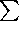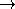#include <CGAL/QP_solution.h>

### Definition

An object of class Quadratic_program_solution<ET> represents the solution of a linear or convex quadratic program of the general form



 (QP) minimize xTDx+cTx+c0 subject to Ax ~ b, lxu

in n real variables x=(x0,...,xn-1).

If D=0, the program is a linear program; if the variable bounds are x0, we have a nonnegative program. Objects of type Quadratic_program_solution<ET> are returned by any of the four functions solve_quadratic_program, solve_linear_program, solve_nonnegative_quadratic_program, and solve_nonnegative_linear_program.

### Example

QP_solver/first_qp.cpp

### Terminology

If there is no x that satisfies all the (in)equalities, the program is called infeasible, otherwise, it is feasible, and any x that satisfies all (in)equalities is called a feasible solution.

If the objective function value becomes arbitrarily small over the feasible region (the set of feasible solutions), the program is called unbounded, and bounded otherwise.

Any program that is both feasible and bounded has at least one feasible solution x* whose objective function value is not larger than that of any other feasible solution. This is called an optimal solution.

Every convex quadratic program (even if it is infeasible or unbounded) has a 'solution' in form of an object of the class Quadratic_program_solution<ET>.

### Types

 Quadratic_program_solution::ET The exact number type that was used to solve the program. Quadratic_program_solution::Variable_value_iterator An iterator type with value type Quotient to go over the values of the variables in the solution. Quadratic_program_solution::Variable_numerator_iterator An iterator type with value type ET to go over the numerators of the variable values with respect to a common denominator. Quadratic_program_solution::Index_iterator An iterator type with value type int to go over the indices of the basic variables and the basic constraints. Quadratic_program_solution::Optimality_certificate_iterator An iterator type with value type Quotient to go over an m-vectorthat proves optimality of the solution. Quadratic_program_solution::Optimality_certificate_numerator_iterator An iterator type with value type ET to go over the numerators of the vectorwith respect to a common denominator. Quadratic_program_solution::Infeasibility_certificate_iterator An iterator type with value type ET to go over an m-vectorthat proves infeasibility of the solution. Quadratic_program_solution::Unboundedness_certificate_iterator An iterator type with value type ET to go over an n-vector w that proves unboundedness of the solution.

### Creation

 Quadratic_program_solution sol; constructs a void instance of Quadratic_program_solution that is associated to no program.

Objects of type Quadratic_program_solution<ET> can be copied and assigned.

Objects of type Quadratic_program_solution<ET> that are associated to an actual program are returned by any of the four functions solve_quadratic_program, solve_linear_program, solve_nonnegative_quadratic_program, and solve_nonnegative_linear_program.

### Example

QP_solver/first_qp.cpp

### Operations

 bool sol.is_void () returns true iff sol is not associated to a program. The condition !sol.is_void() is a precondition for all access methods below.

### Solution status

Here are the access methods for the status of the solution.

 bool sol.is_optimal () returns true iff sol is an optimal solution of the associated program. bool sol.is_infeasible () returns true iff the associated program is infeasible. bool sol.is_unbounded () returns true iff the associated program is unbounded. Quadratic_program_status sol.status () returns the status of the solution; this is one of the values QP_OPTIMAL, QP_INFEASIBLE, and QP_UNBOUNDED, depending on whether the program asociated to sol has an optimal solution, is infeasible, or is unbounded. int sol.number_of_iterations () returns the number of iterations that it took to solve the program asociated to sol.

### Solution values

The actual solution (variable and objective function values) can be accessed as follows.

 Quotient sol.objective_value () returns the objective function value of sol. ET sol.objective_value_numerator () returns the numerator of the objective function value of sol. ET sol.objective_value_denominator () returns the denominator of the objective function value of sol. Variable_value_iterator sol.variable_values_begin () returns a random-access iterator over the values of the variables in sol. The value type is Quotient, and the valid iterator range has length n. Variable_value_iterator sol.variable_values_end () returns the corresponding past-the-end iterator. Variable_numerator_iterator sol.variable_numerators_begin () returns a random-access iterator it over the values of the variables in sol, with respect to a common denominator of all variables. The value type is ET, and the valid iterator range has length n. Variable_numerator_iterator sol.variable_numerators_end () returns the corresponding past-the-end iterator. ET sol.variables_common_denominator () returns the common denominator of the variable values as referred to by the previous two methods.

### Basic variables and constraints

The solution of a linear or quadratic program distinguishes 'important' variables (the ones not attaining one of their bounds), and 'important' constraints (the ones being satisfied with equality). The following methods grant access to them.

 Index_iterator sol.basic_variable_indices_begin () returns a random access iterator over the indices of the basic variables. The value type is int. It is guaranteed that any variable that is not basic in sol attains one of its bounds. In particular, if the bounds are of type x0, all non-basic variables have value 0. Index_iterator sol.basic_variable_indices_end () returns the corresponding past-the-end iterator. int sol.number_of_basic_variables () returns the number of basic variables, equivalently the length of the range determined by the previous two iterators.

### Example

QP_solver/important_variables.cpp

 Index_iterator sol.basic_constraint_indices_begin () returns a random access iterator over the indices of the basic constraints in the system Ax ~ b. The value type is int. It is guaranteed that any basic constraint is satisfied with equality. In particular, if the system is of type Ax=b, all constraints are basic. Index_iterator sol.basic_constraint_indices_end () returns the corresponding past-the-end iterator. int sol.number_of_basic_constraints () returns the number of basic constraint, equivalently the length of the range determined by the previous two iterators.

### Example

QP_solver/first_qp_basic_constraints.cpp

### Output

 template std::ostream& std::ostream& out << sol writes the status of sol to the stream out. In case the status is QP_OPTIMAL, the optimal objective value and the values of the variables at the optimal solution are output as well. For more detailed information about the solution (like basic variables/constraints) please use the dedicated methods of Quadratic_program_solution.

### Validity

The following four methods allow you to check whether sol indeed solves the program that you intended to solve. The methods use the certificates described in the advanced section below and thus save you from validating the certificates yourself (if you believe in the correctness of these methods; otherwise, you can look at their implementation to convince yourself).

By passing a suitable option to the solution function, you can make sure that this check is done automatically after the solution of the program, see Quadratic_program_options. If the check fails, a logfile is generated that contains the details, and an error message is written to std::cerr (see QP_solver/cycling.cpp for an example that uses this option).

 template bool sol.solves_quadratic_program ( QuadraticProgram qp) returns true iff sol solves the quadratic program qp. If the result is false, you can get a message that describes the problem, through the method get_error().

 template bool sol.solves_linear_program ( LinearProgram lp) returns true iff sol solves the linear program lp. If the result is false, you can get a message that describes the problem, through the method get_error().

 template bool sol.solves_nonnegative_quadratic_program ( NonnegativeQuadraticProgram qp) returns true iff sol solves the nonnegative quadratic program qp. If the result is false, you can get a message that describes the problem, through the method get_error().

 template bool sol.solves_nonnegative_linear_program ( NonnegativeLinearProgram lp) returns true iff sol solves the nonnegative linear program lp. If the result is false, you can get a message that describes the problem, through the method get_error().

 bool sol.is_valid () returns false iff the validation through one of the previous four functions has failed. std::string sol.get_error () returns an error message in case any of the previous four validation functions has returned false.advanced### Certificates

A certificate is a vector that admits a simple proof for the correctness of the solution. Any non-void object of Quadratic_program_solution<ET> comes with such a certificate.

Lemma 1 (optimality certificate): A feasible n-vector x* is an optimal solution of (QP) if an m-vectorwith the following properties exist.

1. if the i-th constraint is of type(, respectively), theni0 (i0, respectively).
2.T(Ax*-b) = 0.
3.0, if x*j = lj < uj (cT +T A + 2x*TD)j = 0, if lj < x*j < uj0, if lj < uj = x*j.

Proof: Let x be any feasible solution. We need to prove that

cTx+ xTDxcTx* + x*TDx*.

For this, we argue as follows.



 cTx+ 2x*TDxcTx+ 2x*TDx+T(Ax-b) (by Ax ~ b and 1.) = (cT +T A + 2x*TD)x-Tb(cT +T A + 2x*TD)x* -Tb (by lxu and 3.) = cTx* + 2x*TDx* (by 2.)

After adding xTDx- xTDx- x*TDx* = -x*TDx* to both sides of this inequality, we get

 cTx+ xTDx- (x-x*)TD(x-x*)cTx* + x*TDx*,

and since D is positive semidefinite, we have (x-x*)TD(x-x*)0 and the lemma follows.

Optimality_certificate_iterator sol.optimality_certifcate_begin ()
returns a random access iterator over the optimality certificateas given in Lemma 1, with respect to the solution x* obtained from sol.variable_values_begin(). The value type is Quotient<ET>, and the valid iterator range has length m.
 Precondition: sol.is_optimal()

Optimality_certificate_iterator sol.optimality_certificate_end () returns the corresponding past-the-end iterator.

Optimality_certificate_numerator_iterator
sol.optimality_certifcate_numerators_begin ()
returns a random access iterator over the numerator values of the optimality certificate, with respect to the common denominator returned by sol.variables_common_denominator(). The value type is ET, and the valid iterator range has length m.

Optimality_certificate_numerator_iterator
sol.optimality_certificate_numerators_end ()
returns the corresponding past-the-end iterator.

### Example

QP_solver/optimality_certificate.cpp

Lemma 2 (infeasibility certificate): The program (QP) is infeasible if an m-vectorwith the following properties exist.

1. if the i-th constraint is of type(, respectively), theni0 (i0, respectively).
2.0 if uj=T Aj0 if lj=-.

3.Tb  <j:TAj <0TAj uj   +j:TAj >0TAj lj.

Proof: Let us assume for the purpose of obtaining a contradiction that there is a feasible solution x. Then we get



 0T(Ax-b) (by Ax ~ b and 1.) =j:TAj <0TAj xj   +j:TAj >0TAj xj -T bj:TAj <0TAj uj   +j:TAj >0TAj lj -T b (by lxu and 2.) > 0 (by 3.),

and this is the desired contradiction 0>0.

Infeasibility_certificate_iterator
sol.infeasibility_certificate_begin ()
returns a random access iterator over the infeasibility certificateas given in Lemma 2. The value type is ET, and the valid iterator range has length m.
 Precondition: sol.is_infeasible()

Infeasibility_certificate_iterator
sol.infeasibility_certificate_end ()
returns the corresponding past-the-end iterator.

### Example

QP_solver/infeasibility_certificate.cpp

Lemma 3 (unboundedness certificate:) Let x* be a feasible solution of (QP). The program (QP) is unbounded if an n-vector w with the following properties exist.

1. if the i-th constraint is of type(, =, respectively), then (Aw)i0 ((Aw)i0, (Aw)i=0, respectively).
2.0 if lj is finite wj0 if uj is finite.

3. wTDw=0 and (cT+2x*TD)w<0.

The vector w is called an unbounded direction.

Proof: For a real number t, consider the vector x(t):=x*+tw. By 1. and 2., x(t) is feasible for all t0. The objective function value of x(t) is



 cT x(t) + x(t)TD x(t) = cTx* + tcTw+ x*TDx* + 2tx*TDw+ t2 wTDw = cTx* + x*TDx* + t(cT + 2x*TD)w+ t2wTDw.

By condition 3., this tends to -for t, so the problem is indeed unbounded.

Unboundedness_certificate_iterator
sol.unboundedness_certificate_begin ()
returns a random acess iterator over the unbounded direction w as given in Lemma 3,with respect to the solution x* obtained from sol.variable_values_begin(). The value type is ET, and the valid iterator range has length n.
 Precondition: sol.is_unbounded()

Unboundedness_certificate_iterator
sol.unboundedness_certificate_end ()
returns the corresponding past-the-end iterator.

### Example

QP_solver/unboundedness_certificate.cppadvanced